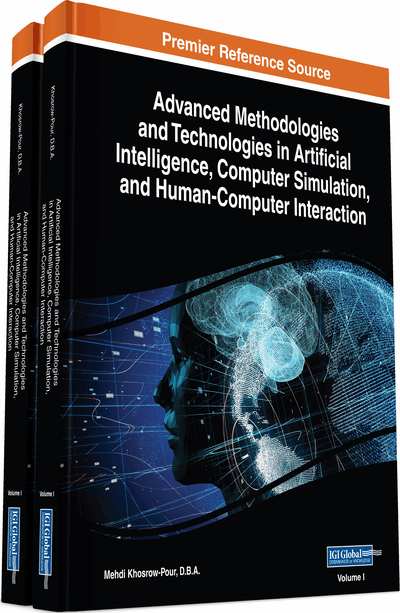# Uniform Random Number Generation With Jumping Facilities

DOI: 10.4018/978-1-5225-7368-5.ch025
OnDemand:
(Individual Chapters)
Available
\$37.50
No Current Special Offers

## Abstract

A facility for generating sequences of pseudorandom numbers is a fundamental part of computer simulation systems. Furthermore, multiple independent streams of random numbers are often required in simulation studies, for instance, to facilitate synchronization for variance-reduction purposes, and for making independent replications. A portable set of software utilities is described for uniform random-number generation. It provides for multiple generators (streams) running simultaneously, and each generator (stream) has its sequence of numbers partitioned into many long disjoint contiguous substreams. Simple procedure calls allow the user to make any generator “jump” ahead/back v steps (random numbers). Implementation issues are discussed. An efficient and portable code is also provided to implement the package. The basic underlying generator CMRG (combined multiple recursive generator) combines two multiple recursive random number generators with a period length of approximately 2191 (≈ 3.1× 1057), good speed, and excellent theoretical properties.
Chapter Preview
Top

## Background

There are a number of methods for generating the random numbers, of which the most popular are the congruential methods (mixed, multiplicative, and additive). The (mixed) linear congruential generators (LCGs) are defined by­x0∈{1,…,m–1} i > 0.where m (the modulus) is a positive integer (usually a very large primary number), a (the multiplier) ∈{1,…,m–1} and c (the increment) is a nonnegative integer. This mathematical notation signifies that xi is the remainder of (axi-1+c) divided by m. Hence, xi∈{1,…,m–1}. Thus, random variable ui is a uniform 0, 1 variable. Note that

.

## Complete Chapter List

Search this Book:
Reset